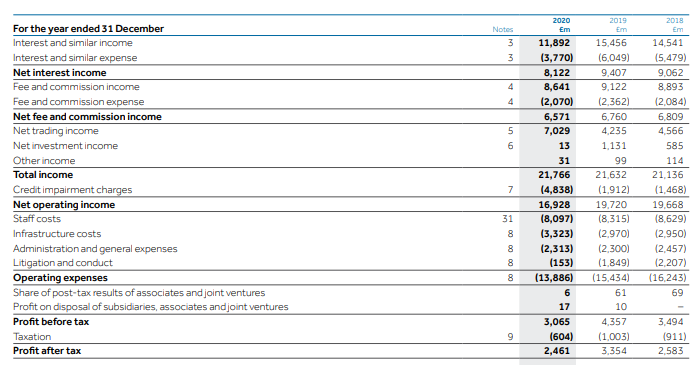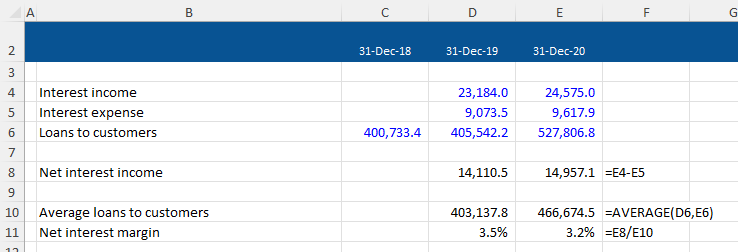## Net Interest Income

Net interest income is used to assess a bank’s profitability. It is calculated by taking the difference between the interest income (generated by the bank’s interest-earning assets) and the interest expense (incurred by the bank’s interest-bearing liabilities).

A bank’s interest-earning assets are primarily the loans it makes to its customers. Interest-bearing liabilities are usually the deposits made by customers plus any borrowings from other banks and the financial markets. Therefore net interest income will depend on the size and mix of the bank’s loan book as well as the bank’s financing mix. It can also be affected by changes in interest rates.

Net interest income can be thought of as the banking equivalent of ‘gross profit’. It measures the profitability of the bank’s core operations before taking into account other business expenses.

Net interest income is used to calculate net interest margin, which is net interest income divided by average interest-earning assets. This allows the bank’s profitability to be analyzed over time or compared with other banks, as it won’t be distorted by differences in the size of the loan book.

Net Interest Income = Interest Income – Interest Expense

## Net Interest Margin

Net interest income can be affected by many factors, including the size and mix of the bank’s loan book, the bank’s financing mix, and current interest rates. Therefore, analysts often calculate net interest margin to help them understand profitability trends for a bank or when conducting peer analysts of banks. Net interest margin is calculated using the following formula:

Net Interest Margin = Net Interest Income for Period/Average Interest Earning Assets for Period

If a bank’s loan book is growing over time or we wish to compare banks with different sizes of the loan book, net interest income alone will be of limited use in understanding a bank’s profitability.

## Key Learning Points

• Net interest income is a key performance metric when assessing a bank’s profitability
• This metric will depend on the bank’s mix of interest-earning assets and interest-bearing liabilities in the balance sheet
• Analysts often divide net interest income by average interest-earning assets to provide a net interest margin. This helps analyze a bank’s profitability over time or to compare a bank’s profitability to its peers

## Where to Find Net Interest Income?

Banks disclose net interest income on the face of their quarterly and annual income statement. It is presented at the top of the income statement, reflecting the fact that it is a core profitability metric.

Below is an extract of a bank’s income statement:Extract from BBank Plc Consolidated Income Statement for the year ended December 31, 2019

-Net interest income is calculated as interest and similar income minus interest and similar expense

-BBank’s net interest income was £9,407m in 2019, which is a 3.8% increase on the net interest income in 2018

-BBank also generates income from fees and commissions, but also incurs credit losses on its loans and has significant operating expenses. Net interest income does not capture these items, so it’s important to remember that it is just one way to measure a bank’s profitability

## Example

Based on the information below, we have been asked to calculate net interest income and net interest margin for this bank in 2019 and 2020.Net interest income is calculated as follows:The net interest income has increased between 2019 and 2020, which means the bank has increased its profitability from its core operations during the year. However, it isn’t clear at this stage what is driving this increased profitability.

We can now calculate net interest margin as follows:The net interest margin has decreased during the year, even though net interest income has increased. This is because the growth rate in average loans to customers in 2020 exceeds the growth rate in net interest income in that year – this suggests that new loans to customers are at lower interest rates than older loans.